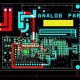# Basic Electrical Topics

In this tutorial we are going to learn about Basic Electrical Topics

1. Basic Electrical Concept
2. Basic Electrical Laws
3. Electrical Circuit Analysis
4. Circuit Theorem
5. Capacitors and Inductors
6. Operational Amplifiers
7. Circuits – First Order
8. Circuits –  Second Order
9. AC Circuit and Phasors
10. Sinusoidal Steady State Analysis
11. AC Power Analysis
12. Three Phase Circuits
13. Magnetic Coupling
14. Frequency Response
15. Laplace Transforms
16. Application of Laplace
17. Fourier Series
18. Fourier Transform
19. Two Port Network
• Basic Electrical Concept – It is like that – current, voltage, resistance, charge, power, efficiency etc.
• Basic Electrical Laws – Basic law in electricity is Ohm’s law is V=IR and here The V is for voltage that means the potential difference between two charges.
• Electrical Circuit Analysis – This is the mathematical analysis of an electrical or electronic circuit. This is the process of studying analyzing electrical quantities through calculations. Through this analysis, we can find the unknown elements of a circuit, such as voltage, current, resistance, impedance, and power, among others, across its component.
• Circuit Theorem – There is difference types of circuit theorem like Superposition, Source Transformations, Thevenin’s Theorem, Norton’s Theorem, Maximum Power Transfer.
• Capacitors and Inductors – The capacitor stores energy in an electric field and an inductor stores energy in a magnetic field
• Operational Amplifiers – Op-amp is a three-terminal device that consists of two high impedance inputs. Here is one of the inputs is called the Inverting Input, marked with a negative or “minus” sign, (–).
• First Order Circuits – It means that circuits which contain only one energy storage element (capacitor or inductor), and that can, therefore, be described using only a first order differential equation.
• Second Order Circuit –The circuit which have characterized by a second-order differential equation and it is consists of resistors and the equivalent of two energy storage elements.
• AC Circuit and Phasors – The voltage phasors  to give the total voltage. In parallel have the same voltage phasor. The current phasors add to give the total current.
• Sinusoidal Steady State Analysis – It is related to the  angular frequency, peak value, RMS value, Frequency and phase of a sinusoidal signal.
• AC Power Analysis – AC power analysis means transformer and AC generator and other related high-voltage devices are required to generate, transmit and distribute alternating voltage and Current.
• Three Phase Circuits – Mostly all electric power generation and most of the power transmission in the world is in the form of three-phase AC circuits.
• Magnetic Coupling – It transfers torque from one shaft to another, but using a magnetic field rather than a physical mechanical connection.
• Frequency Response – Its means the quantitative measure of the output spectrum of a system or device in response to a stimulus, and is used to characterize the dynamics of the system.
• Laplace Transforms – It converts a function of a real variable (often time) to a function of a complex variable. (complex frequency).
• Application of Laplace – It is transforms differential equations into algebraic equations and convolution into multiplication.
• Fourier series –It is a way of representing a periodic function as a (possibly infinite) sum of sine and cosine functions.
• Fourier Transform – It is a Fourier transform is a mathematical transform that decomposes a function into its constituent frequencies.
• Two Port Network – It is the device with two pairs of terminals to connect to external circuits.## About EEE

We have designing Experience for the last 40 years.